### 2019-07-08: Time Series Data Analysis - What, Why and How

In this article, I plan to introduce time series data, and discuss few fundamental, yet important concepts on how time series data is analyzed in the context of data science. In the latter part of the article, I will explain how I conducted time series data analysis on EEG data, and discuss what was achieved from it.Visualization of an EEG time series
If you are new to time series data analysis, the first question is, what is time series data? In layman's terms, it's a set of data points collected over a period of time (hence the term "series"). Each data point represents the state of the observed object(s) at a point in time.

In time series data, the collected data should indicate when each observation was made, along with the observations. These observations could be made at regular intervals or irregular intervals. In most time series data collections, a fixed set of properties are observed at each instance, hence tabular data formats such as CSV are widely used to store time series data.

Now that we introduced what and why of time series data analysis, let's move on to how. How time series data is analyzed depends on the domain of data, but there are few fundamental techniques used for this.

#### What does my data looks like?

Statistical measures provide a good estimate for the majority of data without presenting all data. They come in handy when describing the nature of large sets of data. Statistics such as mean, median and mode indicate the central tendency (read more), while statistics such as minimum and maximum indicate the range of data.

Though the above statistics provide a good estimate of the central tendency and range of the data, it does not describe how densely or sparsely the data is distributed across that range. This is where statistics such as variance and standard deviation comes into the picture. They provide an estimate of how far the majority of data deviates from the mean.

In the context of time series data, these statistics indicate the nature of data observed, and helps to eliminate outliers. But what if the majority of data is not scattered around a center? This could be evaluated by comparing the central tendency estimates (mean, mode and median) with each other. If they deviate largely from each other, it could be an indication of skewed data. Nevertheless, probability density functions could be useful for visualization and estimation in such cases.

#### Signal Processing and Time Series Data

In statistical measures, the relationships between consecutive data points are not taken into consideration. These measures cannot capture how data changes over time, and only provide time-invariant estimates of data. This is where signal processing techniques come in handy for analyzing time series data. Here, the time series data is treated as a signal, and signal processing techniques are applied to eliminate noise (filtering), observe periodic trends (spectral density), and much more.

#### Some Data Points are Missing!

Having missing data points is a rather common issue encountered when performing time series data analysis. When you don't have data at a data point, you have two options: 1) to approximately determine the missing value using available data (interpolation), or 2) to ignore that data point entirely. The latter case cannot be applied if signal processing techniques are to be applied on the data, as it changes the sampling frequency of data.

When analyzing time series data, though the fundamentals are the same, applications may vary from domain to domain. I recently collaborated on a project with Dr. Sampath, and Dr. Mark Jaime at IUPUC to determine if the correlation between Autism Spectrum Disorder (ASD) and Social Interaction can be captured through EEG recordings. We addressed this question by building a set of classifiers that uses EEG data to predict whether or not a subject has ASD.

The preliminary work of collecting and pre-processing EEG data, and using them to build the classifiers is published in the Book Chapter "Electroencephalogram (EEG) for Delineating Objective Measure of Autism Spectrum Disorder" in Computational Models for Biomedical Reasoning and Problem Solving, IGI Global [Link]. Next, we extended this work by adopting an approach that takes both short term and long term trends in EEG data into account. We submitted a paper titled "Analysis of Temporal Relationships between ASD and Brain Activity through EEG and Machine Learning" that elaborates on this work to IEEE Information Reuse and Integration for Data Science Conference (IEEE IRI) 2019 (In Press). I'll discuss these points with more detail in the sections below.

EEG data, being a classic example of time series data, requires certain pre-processing steps to eliminate noise, artifacts and transform into features. For this study, we used the following pre-processing pipeline:Pre-processing Raw EEG Data
• Removing low frequency baseline drifts using a 1 Hz high pass filter.
• Removing 50-60 Hz AC noise
• Bad Channel Rejection using two criteria: 1) flat signal for > 5 s or 2) poor correlation with adjacent channels
• Artifact Subspace Reconstruction (ASR) (read more)
These steps were done using EEGLAB, a MATLAB tool for EEG data processing. The signal we obtained from pre-processing contained a minimal number of noise and artifacts, and hosted the majority of brain signals. We used it as a clean signal source to perform feature extraction.Transforming Clean Signal to a Power Matrix
When extracting features, we followed two approaches: In one approach, we decomposed each signal into a 5 signals corresponding to δ, θ, α, β and γ bands.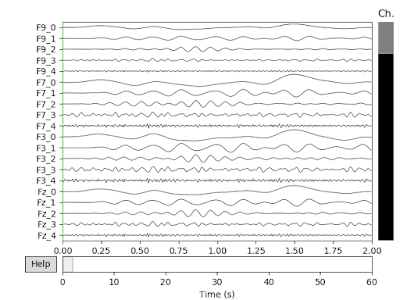EEG signals filtered into Frequency Bands δ, θ, α, β and γ
Next, we chunked the signals into fixed periods of 5 seconds, and calculated the mean, median and mode of each signal. In this manner, we created three series of mean, median and values for each electrode, corresponding to each chunk. We used this as Feature Set I, trained several models using WEKA and documented their evaluation results.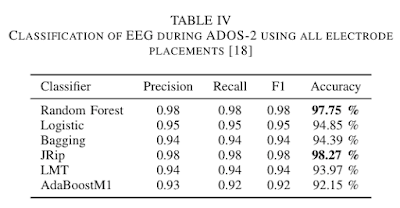Evaluation Results for Feature Set I

The top 6 classifiers show > 90% accuracy when 10 fold cross validation was used. The only features used here were the average and power of the 5 frequency bands of each chunk. The temporal connections between each 5 second chunk was not taken into consideration here.

For our second feature set, we transformed the time series of each electrode into a power matrix by applying wavelet transforms and calculating their spectral densities. Each matrix coefficient indicated the strength of a signal at a given time, and frequency. The diagrams below visualize two power matrices in 2D form.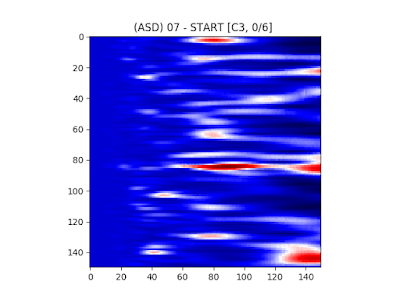Visualization of an Autistic Subject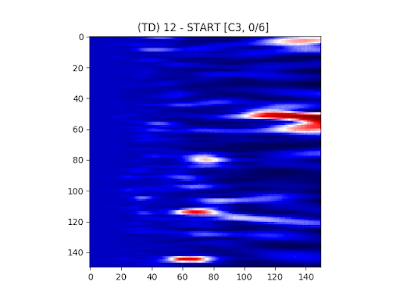Visualization of a Typically Developing Subject

According to the diagrams, the spectral densities show different patterns for ASD and TD subjects for the same electrode. This motivated us to use the spectral density matrices as inputs to a Convolutional Neural Network (CNN), to take temporal trends into account. The architecture of the CNN layers included Dropout, Regularization Kernels, Convolution Layers, Dense Layers and a Sigmoid Neuron for Binary Classification.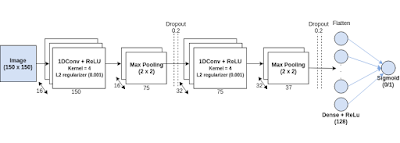Layers of the Convolutional Neural Network (CNN)

Results from both classifiers showed that they perform equally well for the classification task.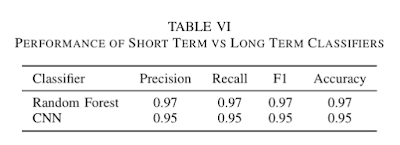Comparing Evaluation Results of Feature Set II with Feature Set I

These metrics were obtained by using a sample size of 8 ASD subjects and 9 TD subjects. Increasing the number of subjects to counter for any class imbalances would more likely result in more generalized evaluation results.

An extended version of this research can be found on arXiv [Link] titled "Electroencephalogram (EEG) for Delineating Objective Measure of Autism Spectrum Disorder (ASD) (Extended Version)".

-- Yasith Jayawardana (@yasithmilinda)